4.1 Supersymmetry

Although initially investigated for other reasons, supersymmetry (SUSY) turns out to have a significant impact on the cosmological constant problem, and may even be said to solve it halfway. SUSY is a spacetime symmetry relating fermions and bosons to each other. Just as ordinary symmetries are associated with conserved charges, supersymmetry is associated with ``supercharges'' Q, whereis a spinor index (for introductions see [131, 132, 133]). As with ordinary symmetries, a theory may be supersymmetric even though a given state is not supersymmetric; a state which is annihilated by the supercharges, Q|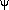> = 0, preserves supersymmetry, while states with Q|>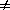0 are said to spontaneously break SUSY.

Let's begin by considering ``globally supersymmetric'' theories, which are defined in flat spacetime (obviously an inadequate setting in which to discuss the cosmological constant, but we have to start somewhere). Unlike most non-gravitational field theories, in supersymmetry the total energy of a state has an absolute meaning; the Hamiltonian is related to the supercharges in a straightforward way: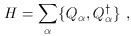(55)

where braces represent the anticommutator. Thus, in a completely supersymmetric state (in which Q|> = 0 for all), the energy vanishes automatically, <|H|> = 0 . More concretely, in a given supersymmetric theory we can explicitly calculate the contributions to the energy from vacuum fluctuations and from the scalar potential V. In the case of vacuum fluctuations, contributions from bosons are exactly canceled by equal and opposite contributions from fermions when supersymmetry is unbroken. Meanwhile, the scalar-field potential in supersymmetric theories takes on a special form; scalar fields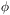i must be complex (to match the degrees of freedom of the fermions), and the potential is derived from a function called the superpotential W(i) which is necessarily holomorphic (written in terms ofi and not its complex conjugate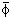i). In the simple Wess-Zumino models of spin-0 and spin-1/2 fields, for example, the scalar potential is given by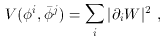(56)

where ðiW = ðW / ði. In such a theory, one can show that SUSY will be unbroken only for values ofi such that ðiW = 0, implying V(i,j) = 0.

So the vacuum energy of a supersymmetric state in a globally supersymmetric theory will vanish. This represents rather less progress than it might appear at first sight, since: 1.) Supersymmetric states manifest a degeneracy in the mass spectrum of bosons and fermions, a feature not apparent in the observed world; and 2.) The above results imply that non-supersymmetric states have a positive-definite vacuum energy. Indeed, in a state where SUSY was broken at an energy scale MSUSY, we would expect a corresponding vacuum energy~ MSUSY4. In the real world, the fact that accelerator experiments have not discovered superpartners for the known particles of the Standard Model implies that MSUSY is of order 103 GeV or higher. Thus, we are left with a discrepancy(57)

Comparison of this discrepancy with the naive discrepancy (54) is the source of the claim that SUSY can solve the cosmological constant problem halfway (at least on a log scale).

As mentioned, however, this analysis is strictly valid only in flat space. In curved spacetime, the global transformations of ordinary supersymmetry are promoted to the position-dependent (gauge) transformations of supergravity. In this context the Hamiltonian and supersymmetry generators play different roles than in flat spacetime, but it is still possible to express the vacuum energy in terms of a scalar field potential V(i,j). In supergravity V depends not only on the superpotential W(i), but also on a ``Kähler potential'' K(i,j), and the Kähler metric Kiconstructed from the Kähler potential by Ki= ð2 K / ði ðj. (The basic role of the Kähler metric is to define the kinetic term for the scalars, which takes the form gµKiðµi ðj.) The scalar potential is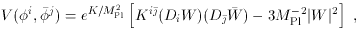(58)

where DiW is the Kähler derivative,Note that, if we take the canonical Kähler metric Ki=i, in the limit MPl ->(G -> 0) the first term in square brackets reduces to the flat-space result (56). But with gravity, in addition to the non-negative first term we find a second term providing a non-positive contribution. Supersymmetry is unbroken when DiW = 0; the effective cosmological constant is thus non-positive. We are therefore free to imagine a scenario in which supersymmetry is broken in exactly the right way, such that the two terms in parentheses cancel to fantastic accuracy, but only at the cost of an unexplained fine-tuning (see for example ). At the same time, supergravity is not by itself a renormalizable quantum theory, and therefore it may not be reasonable to hope that a solution can be found purely within this context.